# Residue Class Rounding

Deutsch

If you do not know what residue classes are, just read on, it will be explained to the extent needed later on.

The concept of rounding seems familiar, but let us try to grab it a little bit more systematically.

Let us assume a set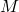of numbers and a subset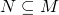of it, whose elements can be represented in the programming or software environment we are having in mind. We want to use a metric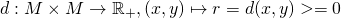. Usually we have three requirements for:

• Identity of indiscernibles: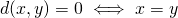• Symmetry: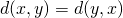• Triangular inquation: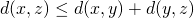Usually the number that we are dealing with can be considered to be within the world of real or complex numbers, we can hence assume that, often evenor if we are honest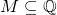. Then we are usually working with, which is kind of implicitly clear. If you do not know complex numbers, just think of real or even rational numbers, which is the most common case anyway. Off course the concepts for rounding of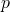-adic numbers are really interesting and beautiful, but since I do not want to explain-adic numbers here, I will not extend on this issue.

What is our intuitive understanding of rounding?
Maybe just a map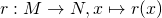,
that is chosen with certain constraints for each x in such a way thatis minimal.
We need the constraints to enforce uniqueness if multiple values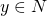with minimalexist. The classical case of rounding to integral numbers has to answer the question of how to round. Ifis a subset of the real numbers, which is „usually“ the case, we have ordering. We can choose between the following constraints:

ROUND_UP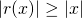Z.B.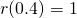and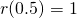and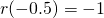ROUND_DOWN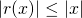Z.B.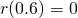and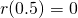and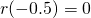ROUND_CEILING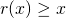Z.B.andandROUND_FLOOR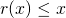Z.B.andandROUND_HALF_UP
Minimize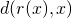, but if multiple optimal values exist for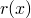, pick the one furthest away from 0, for exampleand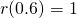andandROUND_HALF_DOWN
Minimize, but if multiple optimal values exist for, pick the one closest to 0, for exampleandandandROUND_HALF_CEILING
Minimize, but if multiple optimal values exist for, pick the largest, for exampleandandandROUND_HALF_FLOOR
Minimize, but if multiple optimal values exist for, pick the smallest, for exampleandandandROUND_HALF_EVEN
Minimize, but if multiple optimal values exist for, pick the one with even last digit. Please observe that this constraint is useful in the classical case, but it cannot be generalized. For example:andandandand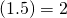ROUND_UNNECESSARY
This constraint does not work in the mathematical sense (or only with ugly abusive math formulas), but programmatically we can do that: We tryand throw an exception if not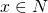.

Usually we are thinking in the decimal system (even though our computers prefer something else, but the computer should serve us and not vice versa..). So we pick a power of ten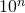with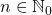, so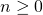. Now we simply define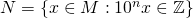,
which means thatcontains all numbers ofwith a maximum of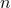digits after the decimal point. This rounding works quite well with something like LongDecimal in Ruby or BigDecimal in Scala or Java, but BigDecimal offers fewer rounding modes than LongDecimal for Ruby.

Now we look at the residue class rounding. We assume such a power of ten. Then we need an integral number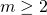and a set of numbers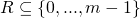, we call them residues. Now we define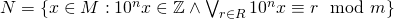.
That means that if we fill the number with zeros to the given number of places after the decimal point, remove the decimal point and perform a division with residue of this number by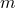, the residue lies in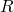. In this case ROUND_HALF_EVEN can become difficult to implement and ambiguous, so we might need to sacrifice it. But even worse, we have to deal with the case that 0 is not inand provide rules how to round 0. The candidates have self-explanatory names:

• ZERO_ROUND_TO_PLUS
• ZERO_ROUND_TO_MINUS
• ZERO_ROUND_TO_CLOSEST_PREFER_PLUS
• ZERO_ROUND_TO_CLOSEST_PREFER_MINUS
• ZERO_ROUND_UNNECESSARY

An important application of this isand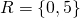. This is used in Switzerland and probably other currency regions for rounding money amounts to multiples of 5 Rappen (0.05 CHF). This has already been described in „Rounding of Money Amounts„. If we haveand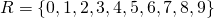we have the usual non-CHF-rounding case. Maybe there are even cases forand. But the concept is more general, if you like to use it.